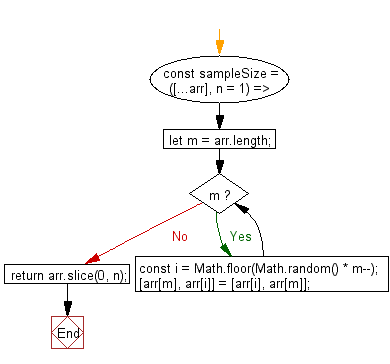# JavaScript: Get n random elements at unique keys from array up to the size of array

## JavaScript fundamental (ES6 Syntax): Exercise-226 with Solution

Write a JavaScript program to get n random elements with unique keys from an array up to the size of the array.

• Shuffle the array using the Fisher-Yates algorithm.
• Use Array.prototype.slice() to get the first n elements.
• Omit the second argument, n, to get only one element at random from the array.

Sample Solution:

JavaScript Code:

``````//#Source https://bit.ly/2neWfJ2
const sampleSize = ([...arr], n = 1) => {
let m = arr.length;
while (m) {
const i = Math.floor(Math.random() * m--);
[arr[m], arr[i]] = [arr[i], arr[m]];
}
return arr.slice(0, n);
};

console.log(sampleSize([1, 2, 3], 2));
console.log(sampleSize([1, 2, 3], 4));
```
```

Sample Output:

```[3,2]
[2,3,1]
```

Pictorial Presentation:Flowchart:Live Demo:

See the Pen javascript-basic-exercise-226-1 by w3resource (@w3resource) on CodePen.

Improve this sample solution and post your code through Disqus

What is the difficulty level of this exercise?

Test your Programming skills with w3resource's quiz.

﻿